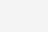# EntityFramework Core 2.0自定义标量函数两种方式

## 自定义标量函数两种方式

（1）函数必须是静态方法且在上下文中声明。

（2）只能作为参数标量值返回。

### 自定义标量函数方式一

```        [DbFunction]
public static string ScalarFunction(string name)
{
throw new NotImplementedException();
}```

### 自定义标量函数方式二

```        public static string ScalarFunction(string name)
{
throw new NotImplementedException();
}```

``` modelBuilder.HasDbFunction(
() => ScalarFunction(null));```

```        [DbFunction(FunctionName = "UdfFunction", Schema = "dbo")]
public static string ScalarFunction(string name)
{
throw new NotImplementedException();
}```

```        public static string ScalarFunction(string name)
{
throw new NotImplementedException();
}

modelBuilder.HasDbFunction(
() => ScalarFunction(null)).HasName("UdfFunction").HasSchema("dbo");
或者
modelBuilder.HasDbFunction(GetType()
.GetMethod("ScalarFunction"), options =>
{
options.HasName("UdfFunction");
options.HasSchema("dbo");
});```

```            using (var context = new EFCoreDbContext())
{
var blogs = context.Blogs
.AsNoTracking();

var result = blogs.Select(b => new BlogDTO()
{
Id = b.Id,
Name = b.Name,
Count = b.Posts.Count() > 0 ? b.Posts.Average(d => d.CommentCount) : 0
}).ToList();
}``````        public static double? UdfAverage(int blogId)
{
throw new Exception();
}```
```  modelBuilder.HasDbFunction(
() => UdfAverage(default(int))).HasSchema("dbo");```

```    public static class AddUdfHelper
{
public static void AddUdfToDatabase(this DbContext context)
{
using (var transaction = context.Database.BeginTransaction())
{
try
{
context.Database.ExecuteSqlCommand(
"IF OBJECT_ID('dbo.UdfAverage', N'FN') IS NOT NULL " +
"DROP FUNCTION dbo.UdfAverage");

context.Database.ExecuteSqlCommand(
"CREATE FUNCTION UdfAverage (@blogId int)" +
@"  RETURNS FLOAT
AS
BEGIN
DECLARE @result AS FLOAT
SELECT @result = AVG(CAST([CommentCount] AS FLOAT)) FROM dbo.Posts AS p
WHERE p.BlogId = @blogId
RETURN @result
END");
transaction.Commit();
}
catch (Exception ex)
{
throw ex;
}
}
}
}``````            using (var context = new EFCoreDbContext())
{
var blogs = context.Blogs
.AsNoTracking();

var result = blogs.Select(b => new BlogDTO()
{
Id = b.Id,
Name = b.Name,
Count = EFCoreDbContext.UdfAverage(b.Id)
}).ToList();
}```